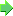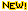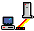## Calculating triangles solver

Here is an Excel ® geometry spreadsheet for the calculation of triangles.

Originally done in my native German, I have translated the important parts- well, sort of.

In this MS Excel ® spreadsheet, triangles can be calculated from three known values.Enter three sides, or two sides and a angle, or two angles and a side, of an arbitrary triangle - and bingo! the missing angles and sides are calculated - along with circumference and area.Without any guarantee, of course. Check if in doubt.

The spreadsheet works well, generally, but with rounding up on two digits. This sometimes causes strange error messages, if more than three values are entered, even if these are apparently correct.Therefore, it is best to always enter three values only. The results should then be reliable.

If a resulting value should turn out so small that it would show only in the third digit behind the comma, displaying "0.00", the unit for the sides can be changed to adapt.Since I don't want unnecessary traffic on this site, it's here for download only as a ZIP-file (16 kb).Now with extra circle calculation spreadsheet.And No Cheating on exams!Download MS Excel spreadsheet for calculating triangles and circle ZIP archiveDownload MS Excel spreadsheet to calculate electric resistors ZIP archive